# Chapter 21 Chemical Reactions Section 1 Chemical Changes

• Slides: 12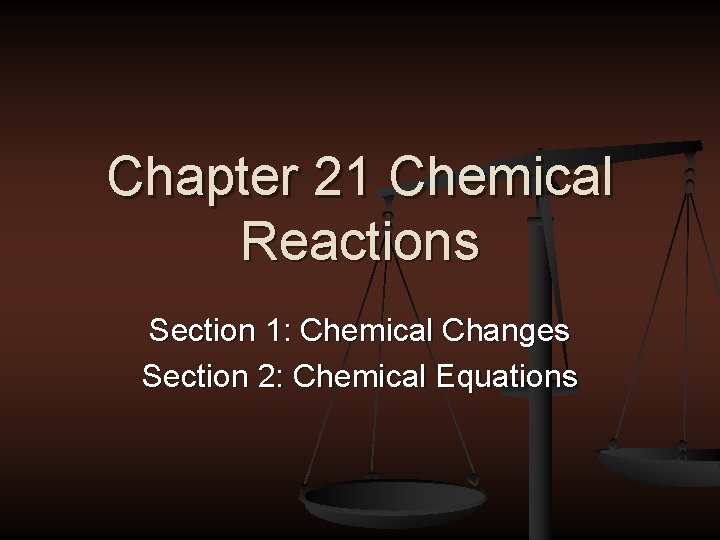Chapter 21 Chemical Reactions Section 1: Chemical Changes Section 2: Chemical Equations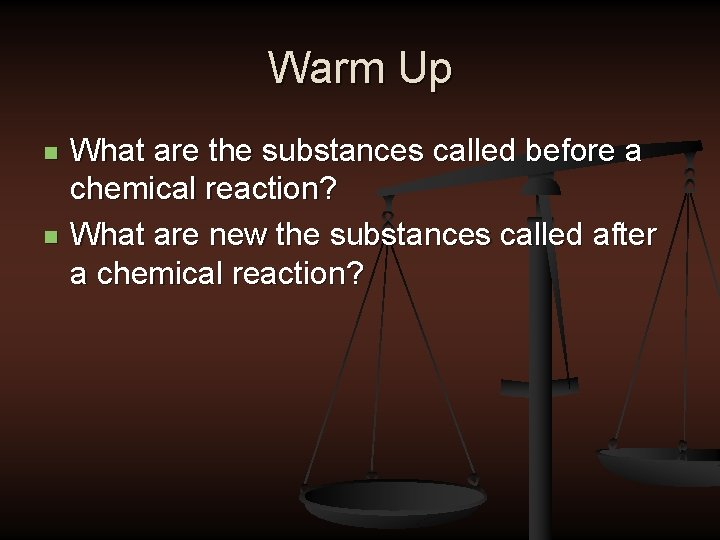Warm Up n n What are the substances called before a chemical reaction? What are new the substances called after a chemical reaction?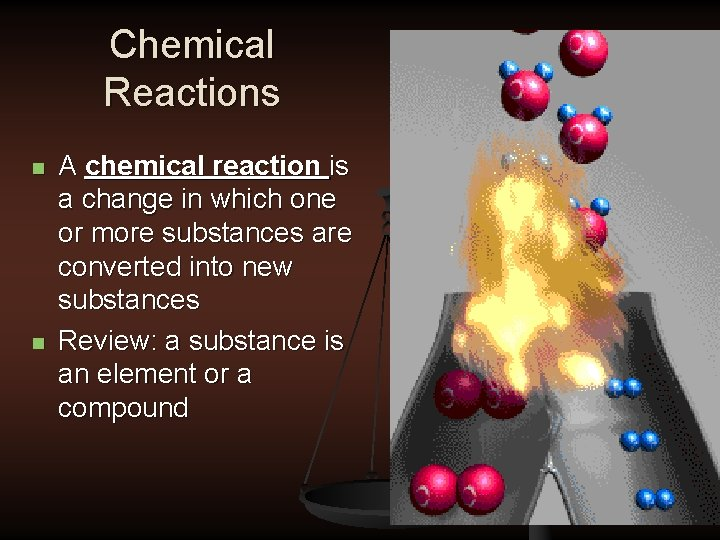Chemical Reactions n n A chemical reaction is a change in which one or more substances are converted into new substances Review: a substance is an element or a compound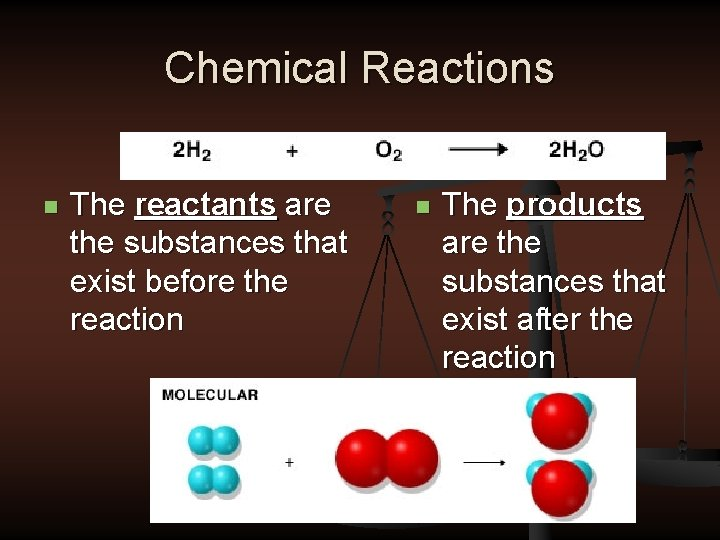Chemical Reactions n The reactants are the substances that exist before the reaction n The products are the substances that exist after the reactionConservation of Mass n n Conservation of Mass: The total mass of the products must always equal the mass of the reactants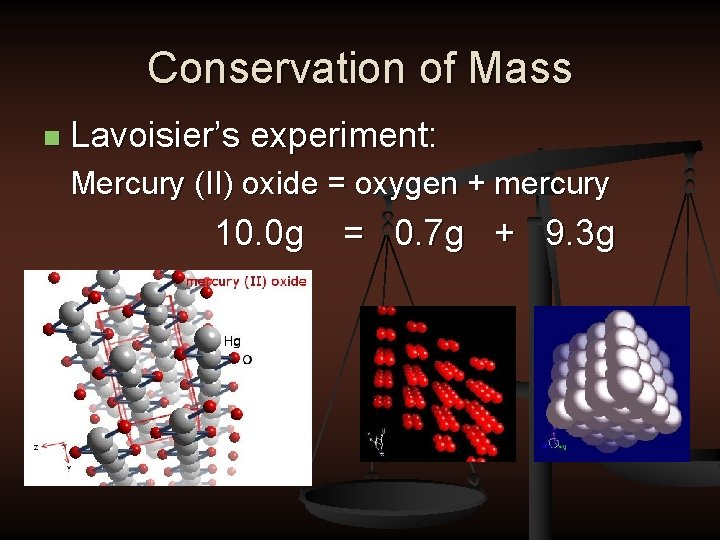Conservation of Mass n Lavoisier’s experiment: Mercury (II) oxide = oxygen + mercury 10. 0 g = 0. 7 g + 9. 3 g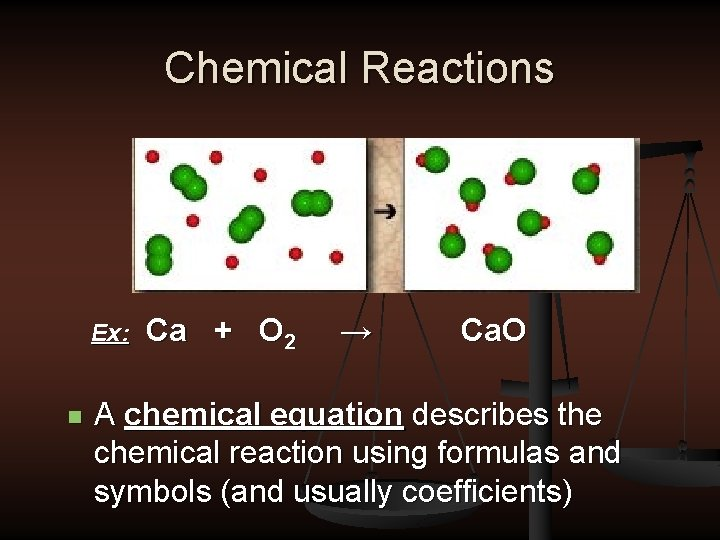Chemical Reactions Ex: n Ca + O 2 → Ca. O A chemical equation describes the chemical reaction using formulas and symbols (and usually coefficients)Chemical Equations Reactants on the Left → stands for “yields” Products on the Right Solids: (s) Liquids: (l) Dissolved in water: (aq) Gases: (g) 2 Mg (s) + O 2 (g) → 2 Mg. O (s)Chemical Reactions n Coefficients represent the number of units of each substance that take part in the reaction Al + O 2 ® Al 2 O 3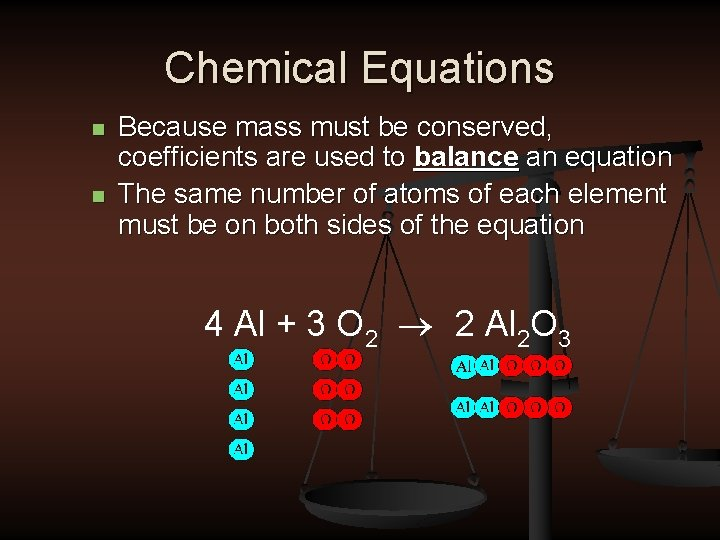Chemical Equations n n Because mass must be conserved, coefficients are used to balance an equation The same number of atoms of each element must be on both sides of the equation 4 Al + 3 O 2 ® 2 Al 2 O 3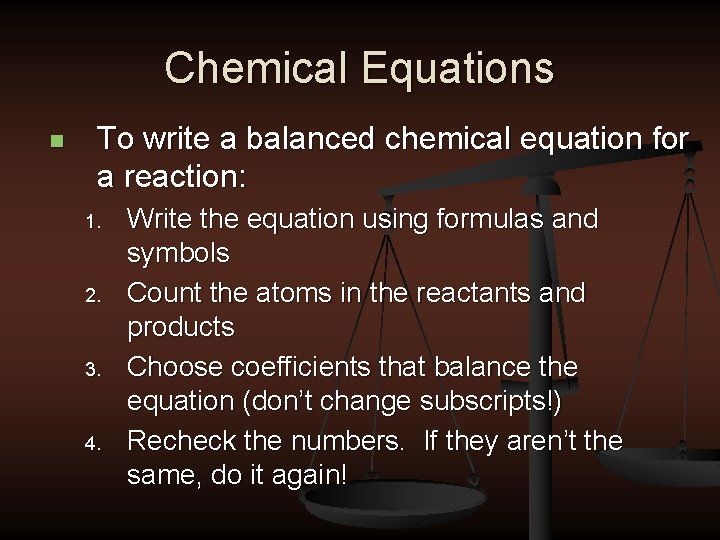Chemical Equations n To write a balanced chemical equation for a reaction: 1. 2. 3. 4. Write the equation using formulas and symbols Count the atoms in the reactants and products Choose coefficients that balance the equation (don’t change subscripts!) Recheck the numbers. If they aren’t the same, do it again!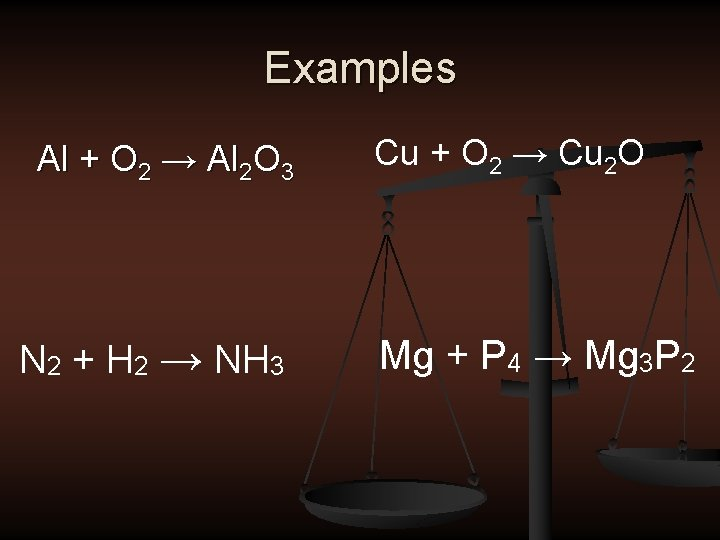Examples Al + O 2 → Al 2 O 3 N 2 + H 2 → NH 3 Cu + O 2 → Cu 2 O Mg + P 4 → Mg 3 P 2